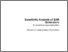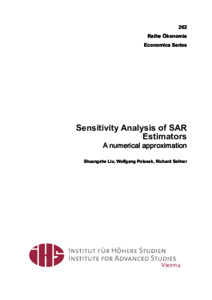# Sensitivity Analysis of SAR Estimators: A numerical approximation

Liu, Shuangzhe; Polasek, Wolfgang and Sellner, Richard (January 2011) Sensitivity Analysis of SAR Estimators: A numerical approximation. Closed Series > Working Paper Series > IHS Economics Series 262Preview
Text
es-262.pdf

Download (427kB) | Preview

## Abstract or Table of Contents

Abstract: Estimators of spatial autoregressive (SAR) models depend in a highly non-linear way on the spatial correlation parameter and least squares (LS) estimators cannot be computed in closed form. We first compare two simple LS estimators by distance and covariance properties and then we study the local sensitivity behavior of these estimators using matrix derivatives. These results allow us to calculate the Taylor approximation of the least squares estimator in the spatial autoregression (SAR) model up to the second order. Using Kantorovich inequalities, we compare the covariance structure of the two estimators and we derive efficiency comparisons by upper bounds. Finally, we demonstrate our approach by an example for GDP and employment in 239 European NUTS2 regions. We find a good approximation behavior of the SAR estimator, evaluated around the non-spatial LS estimators. These results can be used as a basis for diagnostic tools to explore the sensitivity of spatial estimators.;

Item Type: IHS Series 'Spatial autoregressive models' 'Least squares estimators' 'Sensitivity analysis' 'Taylor Approximations' 'Kantorovich inequality' C11, C15, C52, E17, R12 Published 26 Sep 2014 10:39 05 Jul 2019 06:01 1605-7996 https://irihs.ihs.ac.at/id/eprint/2036

### Actions (login required)View Item